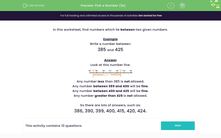# Identify numbers in the range 100-299

In this worksheet, students write a number between two given numbers.Key stage:  KS 2

Curriculum topic:   Number: Number and Place Value

Curriculum subtopic:   Order/Compare Numbers to 1000

Difficulty level:#### Worksheet Overview

In this activity, we will be learning about numbers that lie between two given numbers.

Let's think about numbers which lie between 125 and 185

Sometimes it is useful to imagine the numbers on a number line, like this.This number line helps us to imagine some of the numbers which will fall between 125 and 185.

Ben has written a set of numbers between 125 and 185, but he has got one wrong.

Can you spot it?

124, 126, 158, 184

Ben has written the number 124. This is actually smaller than 125, so should not be included in the set.

Let's try an example.

Example

Which of the following numbers does not fall between 110 and 135?

112, 102, 120, 129, 133.